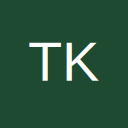# Day 1 A Beautiful Egyptian

• 156|-164

• Okay, so obviously A = 5. As for B and C, it gets a little more tricky.
CC6+6=CBB
So the second digit and third digit have to be the same. Let's work on the third digit since it's the easiest : 6+6=12, we put the 2 in there (So B = 2, that becomes a fact). Now we need to have 2 be in the second digit aswell while having both Cs set to 1 Value. This gives us :

CC6+6=CBB
116+6=122

So A = 5
B = 2
C = 1.

Coordinates are (52|1).
S : South
W : West
E : East.

Now, the way travian works is North-East is (+|+), South West is (-|-), South-East is (+|-) and North-West is (-|+)

So we start at 52|1 (we're at north-east effectively)
We go south by 57 Fields : 52|-56
East by 73 Fields : 125|-56
South by 47 Fields : 125|-103
East by 57 Fields : 182|-103
South by 43 Fields : 182|-146
West by 26 Fields : 156|-146
South by 18 Fields : 156|164

The Coordinates are (156|164)Tell me if my numbers are wrong• (156|-163)

• 156|-163
MissFit COM80

• 156/-164

A=5
B=2
c=1

ign - Frodo Baggins
server- ts20.travian.com

• I forgot mentioning server: ts29

• Should i post answer here?com83(beta): rastogi2008

com29(NYS5x): Slavefactory

Eye halve a spelling chequer, it came with my pea sea.
It plainly marques, four my revue, miss steaks eye kin knot sea.
Eye strike a quay and type a word And weight four it two say
weather eye am wrong oar write It shows me strait a weigh.

• AA3+2=AAA...3+2=A...therefore A=5...553+2=555
CC6+6=CBB...6+6=12...1(2)...therefore B=2...116+6=122...therefore C=1
(AB|C) ->S57->E73->S47->E57->S43->W26->S18->?...starting point (52|1)
Go South 57 fields + 47 Fields +43 fields +18 fields = 165 fields...therefore 1-165=-164
Go East 73 fields + 57 fields = 130 fields and West 26 fields...therefore effectively in total East for 104 fields...therefore 52+104=156

Hence (156|-164)

• 156|-164
Dragun COM2

• (-52|-164)

• How do we know we got the right coordinates is there something marked on the map or do we wait for the second day.

• trompette1996, server COM2, ts2